# Problem 57507. Easy Sequences 88: Double Summation of a Combinatorial Function

Given two positive integers a and b,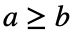, we define the function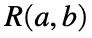as the sum of the reciprocals of the products of all combinations of 1 to a taken b at a time. In Matlab we say that: R = sum(1./prod(nchoosek(a,b)')). For example if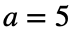and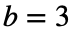:
>> R = sum(1./prod(nchoosek(1:5,3)'))
R =
0.7083
Given a positive integer x, we are asked to evaluate the following double summation:For example for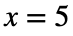:In which, in Matlab, evaluates to:
>> S = sum(arrayfun(@(i) sum(1./prod(nchoosek(1:3,i)')),2:2))+sum(arrayfun(@(i) sum(1./prod(nchoosek(1:4,i)')),2:3))+sum(arrayfun(@(i) sum(1./prod(nchoosek(1:5,i)')),2:4))
S =
5.5833
Please round-off S to the nearest whole number. Therefore, the final output for the above example is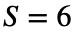.

### Solution Stats

100.0% Correct | 0.0% Incorrect
Last Solution submitted on Jan 10, 2023

### Community Treasure Hunt

Find the treasures in MATLAB Central and discover how the community can help you!

Start Hunting!